# 史上最详细的Yolov3边框预测分析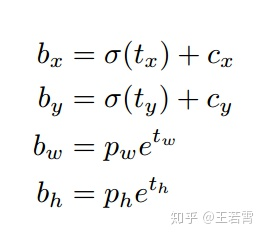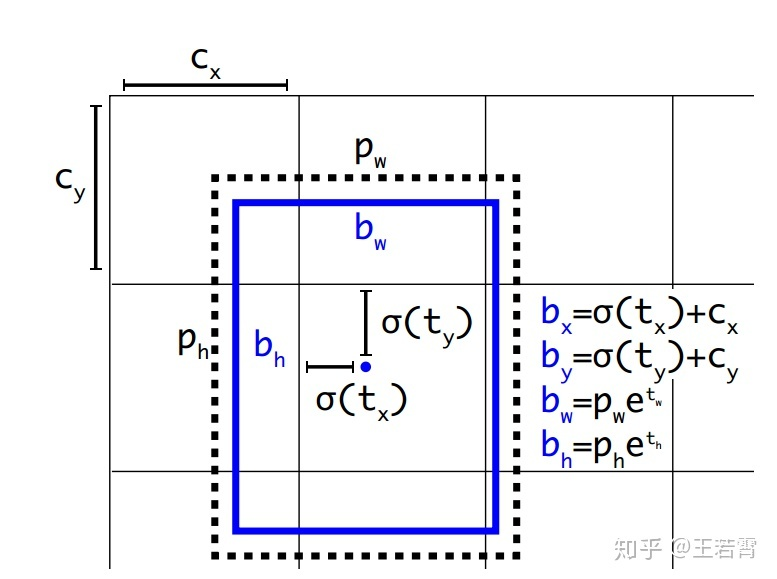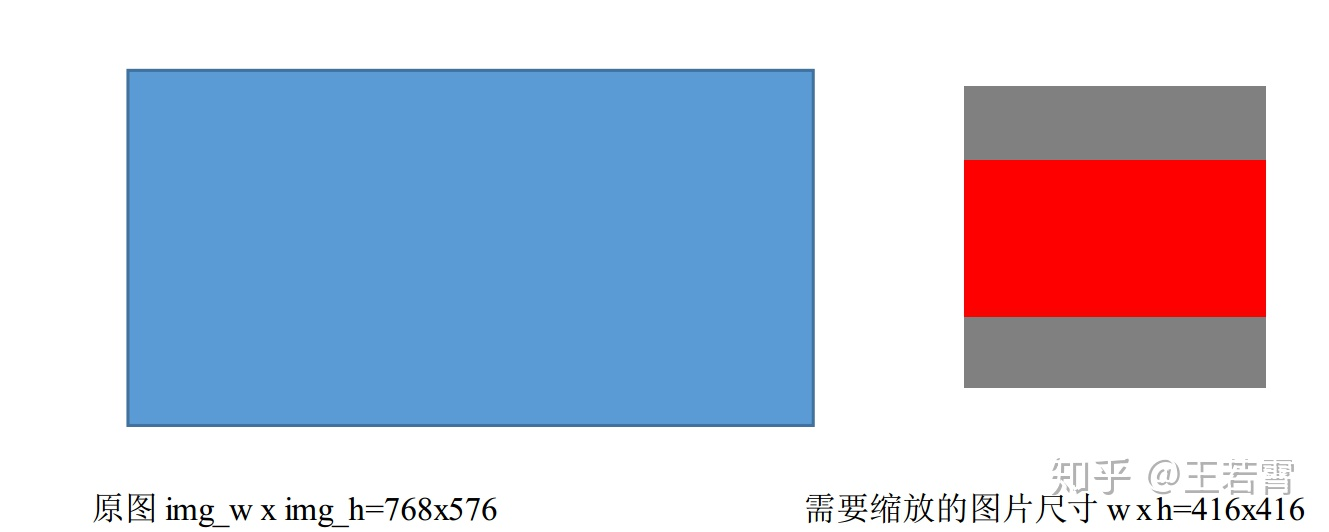def letterbox_image(img, inp_dim):
"""
lteerbox_image()将图片按照纵横比进行缩放，将空白部分用(128,128,128)填充,调整图像尺寸
具体而言,此时某个边正好可以等于目标长度,另一边小于等于目标长度
将缩放后的数据拷贝到画布中心,返回完成缩放
"""
img_w, img_h = img.shape, img.shape
w, h = inp_dim#inp_dim是需要resize的尺寸（如416*416）
# 取min(w/img_w, h/img_h)这个比例来缩放，缩放后的尺寸为new_w, new_h,即保证较长的边缩放后正好等于目标长度(需要的尺寸)，另一边的尺寸缩放后还没有填充满.
new_w = int(img_w * min(w/img_w, h/img_h))
new_h = int(img_h * min(w/img_w, h/img_h))
resized_image = cv2.resize(img, (new_w,new_h), interpolation = cv2.INTER_CUBIC) #将图片按照纵横比不变来缩放为new_w x new_h，768 x 576的图片缩放成416x312.,用了双三次插值
# 创建一个画布, 将resized_image数据拷贝到画布中心。
canvas = np.full((inp_dim, inp_dim, 3), 128)#生成一个我们最终需要的图片尺寸hxwx3的array,这里生成416x416x3的array,每个元素值为128
# 将wxhx3的array中对应new_wxnew_hx3的部分(这两个部分的中心应该对齐)赋值为刚刚由原图缩放得到的数组,得到最终缩放后图片
canvas[(h-new_h)//2:(h-new_h)//2 + new_h,(w-new_w)//2:(w-new_w)//2 + new_w,  :] = resized_image

return canvas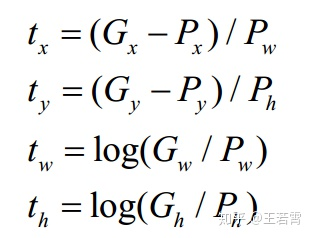$t_w$$t_h$的公式yolov3和faster-rcnn系列是一样的，是物体所在边框的长宽和anchor box长宽之间的比率，不管Faster-RCNN还是YOLO，都不是直接回归bounding box的长宽而是 尺度缩放到对数空间，是怕训练会带来不稳定的梯度。 因为如果不做变换，直接预测相对形变$t_w$$t_h$，那么要求$t_w,t_h>0$，因为你的框的宽高不可能是负数。这样，是在做一个有不等式条件约束的优化问题，没法直接用SGD来做。所以先取一个对数变换，将其不等式约束去掉，就可以了。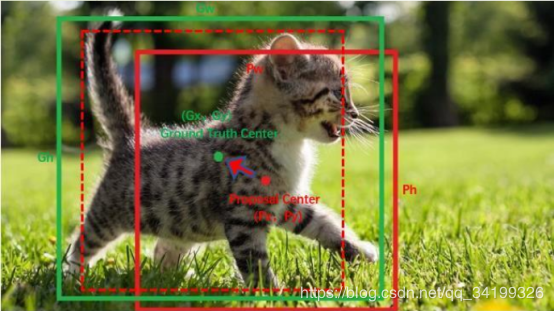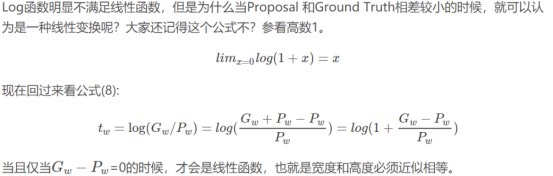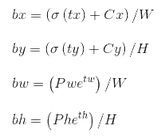box get_yolo_box(float *x, float *biases, int n, int index, int i, int j, int lw, int lh, int w, int h, int stride)
{
box b;
b.x = (i + x[index + 0*stride]) / lw;
// 此处相当于知道了X的index,要找Y的index,向后偏移l.w*l.h个索引
b.y = (j + x[index + 1*stride]) / lh;
b.w = exp(x[index + 2*stride]) * biases[2*n]   / w;
b.h = exp(x[index + 3*stride]) * biases[2*n+1] / h;
return b;
}

float delta_yolo_box(box truth, float *x, float *biases, int n, int index, int i, int j, int lw, int lh, int w, int h, float *delta, float scale, int stride)
{
box pred = get_yolo_box(x, biases, n, index, i, j, lw, lh, w, h, stride);
float iou = box_iou(pred, truth);

float tx = (truth.x*lw - i);
float ty = (truth.y*lh - j);
float tw = log(truth.w*w / biases[2*n]);
float th = log(truth.h*h / biases[2*n + 1]);
//scale = 2 - groundtruth.w * groundtruth.h
delta[index + 0*stride] = scale * (tx - x[index + 0*stride]);
delta[index + 1*stride] = scale * (ty - x[index + 1*stride]);
delta[index + 2*stride] = scale * (tw - x[index + 2*stride]);
delta[index + 3*stride] = scale * (th - x[index + 3*stride]);
return iou;
}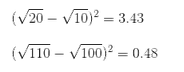#scaling_factor*img_w和scaling_factor*img_h是图片按照纵横比不变进行缩放后的图片，即原图是768x576按照纵横比长边不变缩放到了416*372。
#经坐标换算,得到的坐标还是在输入网络的图片(416x416)坐标系下的绝对坐标，但是此时已经是相对于416*372这个区域的坐标了，而不再相对于(0,0)原点。
output[:,[1,3]] -= (inp_dim - scaling_factor*im_dim_list[:,0].view(-1,1))/2#x1=x1−(416−scaling_factor*img_w)/2,x2=x2-(416−scaling_factor*img_w)/2
output[:,[2,4]] -= (inp_dim - scaling_factor*im_dim_list[:,1].view(-1,1))/2#y1=y1-(416−scaling_factor*img_h)/2,y2=y2-(416−scaling_factor*img_h)/2


void correct_yolo_boxes(detection *dets, int n, int w, int h, int netw, int neth, int relative)
{
int i;
// 此处new_w表示输入图片经压缩后在网络输入大小的letter_box中的width,new_h表示在letter_box中的height,
// 以1280*720的输入图片为例，在进行letter_box的过程中，原图经resize后的width为416， 那么resize后的对应height为720*416/1280,
//所以height为234，而超过234的上下空余部分在作为网络输入之前填充了128，new_h=234
int new_w=0;
int new_h=0;
// 如果w>h说明resize的时候是以width/图像的width为resize比例的，先得到中间图的width,再根据比例得到height
if (((float)netw/w) < ((float)neth/h)) {
new_w = netw;
new_h = (h * netw)/w;
} else {
new_h = neth;
new_w = (w * neth)/h;
}
for (i = 0; i < n; ++i){
box b = dets[i].bbox;
// 此处的公式很不好理解还是接着上面的例子，现有new_w=416,new_h=234,因为resize是以w为长边压缩的
// 所以x相对于width的比例不变，而b.y表示y相对于图像高度的比例，在进行这一步的转化之前，b.y表示
// 的是预测框的y坐标相对于网络height的比值，要转化到相对于letter_box中图像的height的比值时，需要先
// 计算出y在letter_box中的相对坐标，即(b.y - (neth - new_h)/2./neth)，再除以比例
b.x =  (b.x - (netw - new_w)/2./netw) / ((float)new_w/netw);
b.y =  (b.y - (neth - new_h)/2./neth) / ((float)new_h/neth);
b.w *= (float)netw/new_w;
b.h *= (float)neth/new_h;
if(!relative){
b.x *= w;
b.w *= w;
b.y *= h;
b.h *= h;
}
dets[i].bbox = b;
}
}


！！！！！至此总结一下，我们得以知道，原来网络中通过feature map学习到的位置信息是偏移量tx,ty,tw,th，就是在Yolo检测层中，也就是最后的feture map，维度为(batch_size, num_anchors*bbox_attrs, grid_size, grid_size)，对于每张图就是（num_anchors*bbox_attrs, grid_size, grid_size)对于coco的80类，bbox_attrs就是80+5,5表示网络中学习到的参数tx,ty,tw,th，以及是否有目标的score。也就是对于3层预测层，最深层是255*13*13，255是channel，物理意义表征bbox_attrs×3，3是anchor个数。为了计算loss，输出特征图需要变换为(batch_size, grid_size*grid_size*num_anchors, 5+类别数量)的tensor，这里的5就已经是通过之前详细阐述的边框预测公式转换完的结果，即bx,by,bw,bh.对于尺寸为416*416的图像，通过三个检测层检测后，有[(52*52)+(26*26)+(13*13)]*3=10647个预测框，也就是维度为(batchsize,10647,85).然后可以转为x1,y1,x2,y2来算iou,通过score滤去和执行nms去掉绝大多数多余的框，计算loss等操作了。

Pr(Classi|Object) ∗ Pr⁡(Object) ∗ IOU(pred,ground truth) = Pr(Classi) ∗ IOU(pred,ground truth)